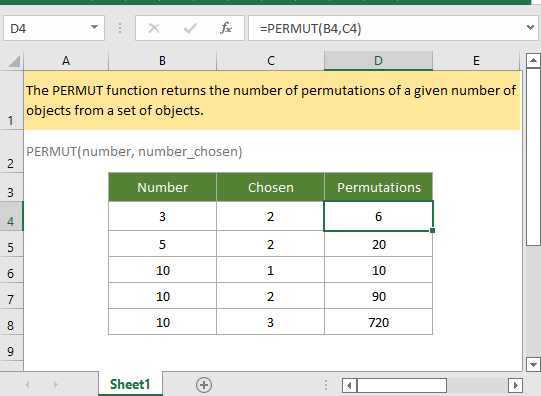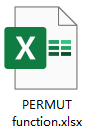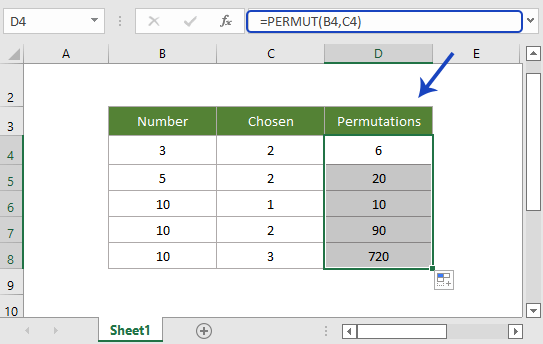## Excel PERMUT Function### Description

The Excel PERMUT function returns the number of permutations of a given number of objects from a set of objects. The PERMUT function does not allow repetitions.

### Syntax and arguments

Formula syntax

PERMUT(number, number_chosen)

Arguments

 Number: Required, an integer that describes the total number of objects. Number_chosen: Required, an integer that describes the number of objects selected from the total objects.

Return Value

The PERMUT function returns an integer value that indicates the total number of permutations.

Remark

1. Any of the arguments are supplied as decimal values, they would be truncated to integers by PERMUT function.

2. The difference between PERTMUT function and PERMUTATION function:

• PERMUT function does not allow repetitions. For example, in a set of 1, 2, 3, to get the permutations of 2 objects from the set, the PERMUT function returns 6 combinations: 12,13,21,23,31,32).
• PERMUTATIONA function does allow repetitions. For example, in a set of 1, 2, 3, to get the permutations of 2 objects from the set, the PERMUTATION function returns 9 combinations: 11,12,13,21,22,23,31,32,33).
• For PERMUT function, the order of the objects matters

3. The equation for PERMUT functions is:Error

1. If any of arguments are non-numeric values, the PERMUT function returns #VALLUE! error value.

2. The PERMUT function returns #NUM! error value,

• if argument number is smaller that argument number_chosen;
• if argument number <= 0, or argument number_chose < 0.

4. If the significance < 1, the PERCENTRANK.INC function returns #NUM! error.

Version

Excel 2003 and later

### Usage and Examples

Example: Basic Usage

In range B4:C9, list the numbers of objects and the number for chosen in each set, to get the number of permutations of each set, please use formula as this:

=PERMUT(B4,C4)

Or directly type the numbers as arguments in formula

=PERMUT(3,2)

Press Enter key to get the first result, then drag autofill handle down to get all results.#### Relative Functions:

• Excel PERCENTRANK Function
The Excel PERCENTRANK function returns the rank of a value in a data set as a percentage of the data set.

• Excel PERCENTRANK.EXC Function
The Excel PERCENTRANK.EXC returns the rank of a value in a data set as a percentage (excludes 0 and 1) of the data set.

• Excel PERCENTRANK.INC Function
The Excel PERCENTRANK.INC function returns the rank of a value in a data set as a percentage (includes 0 and 1) of the data set.

### The Best Office Productivity Tools

#### Kutools for Excel - Helps You To Stand Out From Crowd

 Popular Features: Find/Highlight/Identify Duplicates   |  Delete Blank Rows   |  Combine Columns or Cells without Losing Data   |   Round without Formula ... Super Lookup: Multiple Criteria VLookup | Multiple Value VLookup |  VLookup Across Multi-Sheets |  Fuzzy Lookup... Advanced Drop-down List: Easy Drop Down List   |  Dependent Drop Down List   |  Multi-select Drop Down List .... Column Manager: Add a Specific Number of Columns   |   Move Columns   |   Unhide Columns   |   Compare Columns to Select Same & Different Cells ... Featured Features: Grid Focus   |  Design View   |   Big Formula Bar   |  Workbook & Sheet Manager   |  Resource Library (Auto Text)   |  Date Picker   |  Combine Worksheets   |  Encrypt/Decrypt Cells   |  Send Emails by List   |  Super Filter   |   Special Filter (filter bold/italic/strikethrough...) ... Top 15 Toolset:  12 Text Tools (Add Text, Remove Characters ...)   |   50+ Chart Types (Gantt Chart ...)   |   40+ Practical Formulas (Calculate age based on birthday ...)   |   19 Insertion Tools (Insert QR Code, Insert Picture from Path ...)   |   12 Conversion Tools (Numbers to Words, Currency Conversion ...)   |   7 Merge & Split Tools (Advanced Combine Rows, Split Cells ...)   |   Many More...

Kutools for Excel boasts over 300 features, ensuring that what you need is just a click away...

Supports Office/Excel 2007-2021 & newer, including 365   |   Available in 44 languages   |    Full-featured 30-day free trial.#### Office Tab - Enable Tabbed Reading and Editing in Microsoft Office (include Excel)

• One second to switch between dozens of open documents!
• Reduce hundreds of mouse clicks for you every day, say goodbye to mouse hand.
• Increases your productivity by 50% when viewing and editing multiple documents.
• Brings Efficient Tabs to Office (include Excel), Just Like Chrome, Firefox, And New Internet Explorer.